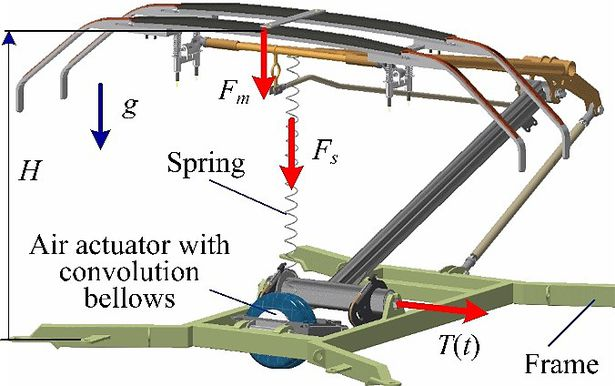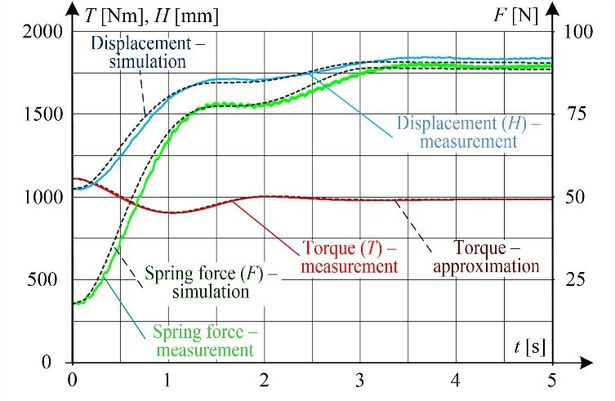# Measurements of raising of 160EC pantograph type - Open Research Data - MOST Wiedzy

## Measurements of raising of 160EC pantograph type### Description

In this description the results of the experiment and also simulation performed on the total assembly of the 160 EC pantograph type is given. Multibody dynamics of pantograph rising due to external torque and forces are measured for parameter validation of the pantograph model.

At a given initial time t = 0, the pantograph head position height was H0 = 1.04 m. Along with the force of gravity, the following forces and torques acted on its arms (Figure): torque T0 = 1110 Nm generated by the pneumatic drive to lift the pantograph arms, force F= 18 N coming from the external spring and used for lowering the pantograph arms, and force F= 78.5 N coming from an additional mass suspended from the pantograph head unit and used to lower its arms.

After sudden removal of force Fm (cutting off the weight of mass m = 8 kg), the transient stage began, which lasted less than 5 s. The steady-state parameter values were as follows: = 1.84 m, = 986 Nm, F= 89.5 N, F= 0. The quantities measured during the laboratory test were H, T, and Fs. The waveforms of these parameters from measurement and simulation are shown in Figure.

For the simulation purpose, the measured torque T(t) was approximated using an analytical function. As can be seen in Figure, this approximation correctly maps the measured torque waveform.

The structure of the pneumatic drive unit is complex. Along with the pneumatic drive, it includes the pressure and air flow control system. This unit was modelled using a simple model consisting of an equivalent spring and an equivalent damper. In the simulation process, the spring and damper parameters are additional unknown parameters. Their values were determined iteratively. The value of the elasticity coefficient kb was assumed constant, while the damping coefficient cb was expressed by a nonlinear function dependent on the speed of pneumatic drive piston rod. This model ensured good matching of simulation results to measurements. More accurate assessment of detailed parameters of individual components of the pneumatic drive model would require taking into consideration the model of its control system. This problem requires further, more advanced research.Test of the complete pantograph: forces and torque acting on pantograph (on the left ); simulation and experimental results (on the right)

### Dataset file

Pantograph_Raising_160EC_Data.xlsx

### Details

Year of publication:
2020
Creation date:
2019
Dataset language:
English
Fields of science:
• Automation, electronic and electrical engineering (Engineering and Technology)
CC BY